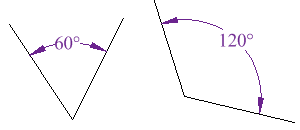ANGLE-

In geometry, an angle is the figure formed by two rays, called the sides of the angle, sharing a common endpoint, called the vertex of the angle. Angles are usually presumed to be in a Euclidean plane or in the Euclidean space, but are also defined in non-Euclidean geometries. In particular, in spherical geometry, the spherical angles are defined, using arcs of great circles instead of rays.

Angle is also used to designate the measure of an angle or of a rotation. This measure is the ratio of the length of a circular arc to its radius. In the case of a geometric angle, the arc is centered at the vertex and delimited by the sides. In the case of a rotation, the arc is centered at the center of the rotation and delimited by any other point and its image by the rotation.

LINEAR PAIR-

Linear Pair of angles From Latin: linearis - "belonging to a line"Definition: Two angles that are adjacent (share a leg) and supplementary (add up to 180)Tr

In the figure above, the two angles JKM and LKM form a linear pair. They are supplementary because they always add to 180 and because they are adjacent, the two non-common legs form a straight line segment JKL.

Two angles are Adjacent if they have a common side and a common vertex (corner point) and don't overlap.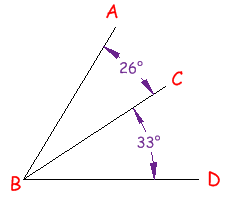Angle ABC is adjacent to angle CBD

Because:

• they have a common side (line CB)
• they have a common vertex (point B)
What Is and Isn't an Adjacent Angle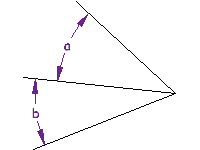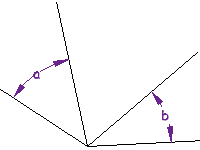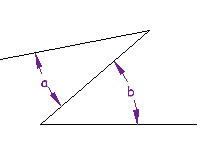they share a vertex and a side

they only share a vertex, not a side

they only share a side, not a vertex

Don't Overlap!

ALSO the angles must not overlap.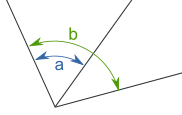angles a and b overlap

COMPLEMENTARY ANGLES-

Two Angles are Complementary if they add up to 90 degrees (a Right Angle).

These two angles (40 and 50) are Complementary Angles, because they add up to 90.

Notice that together they make a right angle.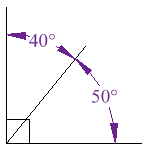But the angles don't have to be together.

These two are complementary because 27 + 63 = 90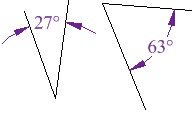SUPPLEMENTARY ANGLES-

Two Angles are Supplementary if they add up to 180 degrees.

These two angles (140 and 40) are Supplementary Angles, because they add up to 180.

Notice that together they make a straight angle.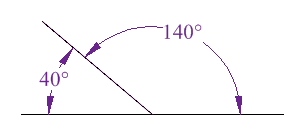But the angles don't have to be together.

These two are supplementary because 60 + 120 = 180